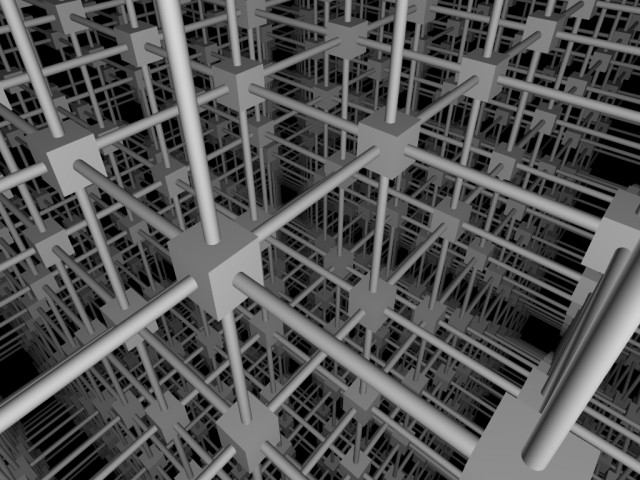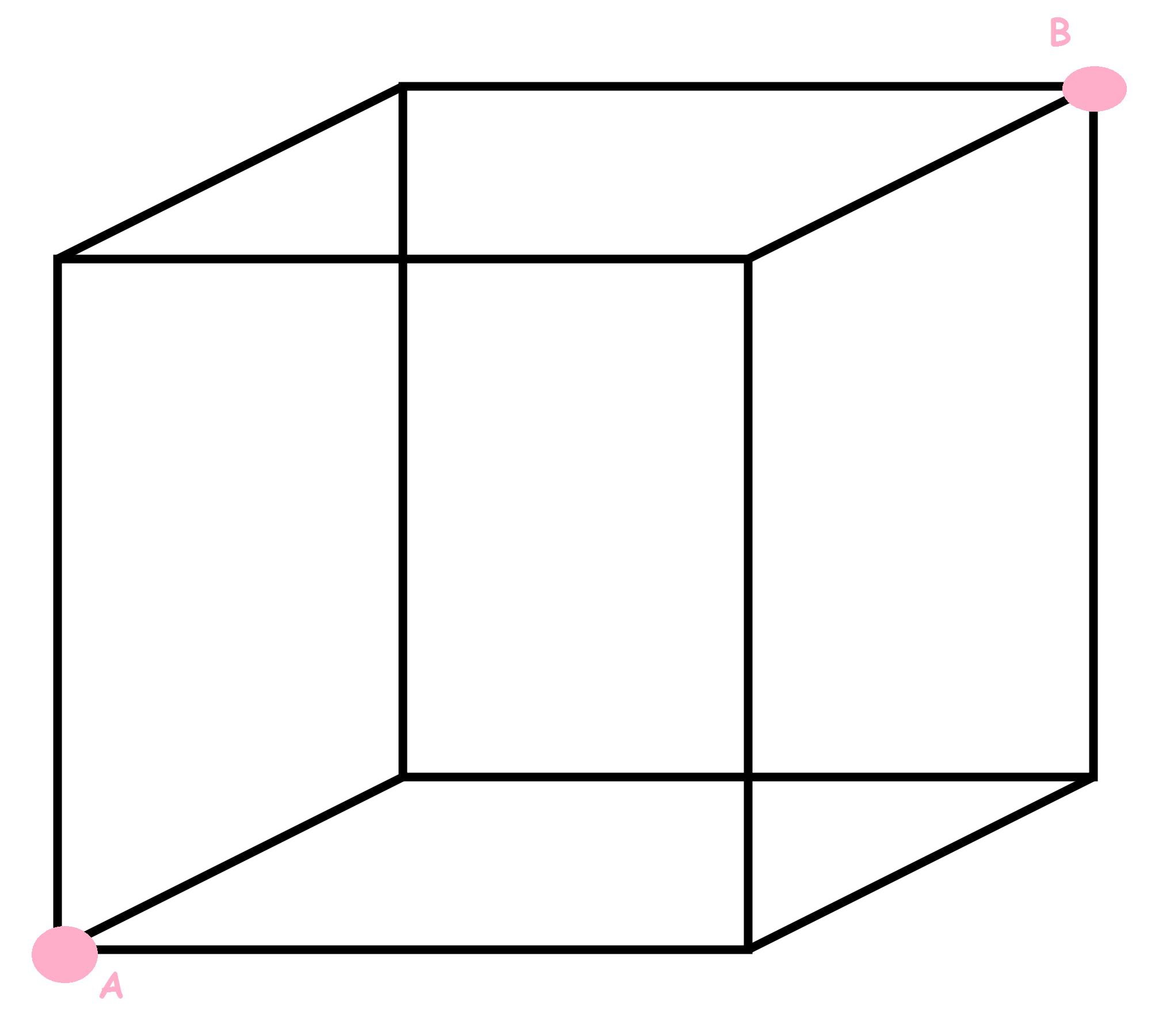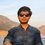# Infinite cubic grid of resisrors

Can we find net resistance between the body diagonal points of a infinite cubic lattice?

The objective is to find net resistance between A & B of the given cube which is part of infinite grid. Let the resistance between any two adjacent vertices is RNote by Pranjal Prashant
5 years, 10 months ago

This discussion board is a place to discuss our Daily Challenges and the math and science related to those challenges. Explanations are more than just a solution — they should explain the steps and thinking strategies that you used to obtain the solution. Comments should further the discussion of math and science.

When posting on Brilliant:

• Use the emojis to react to an explanation, whether you're congratulating a job well done , or just really confused .
• Ask specific questions about the challenge or the steps in somebody's explanation. Well-posed questions can add a lot to the discussion, but posting "I don't understand!" doesn't help anyone.
• Try to contribute something new to the discussion, whether it is an extension, generalization or other idea related to the challenge.

MarkdownAppears as
*italics* or _italics_ italics
**bold** or __bold__ bold
- bulleted- list
• bulleted
• list
1. numbered2. list
1. numbered
2. list
Note: you must add a full line of space before and after lists for them to show up correctly
paragraph 1paragraph 2

paragraph 1

paragraph 2

[example link](https://brilliant.org)example link
> This is a quote
This is a quote
    # I indented these lines
# 4 spaces, and now they show
# up as a code block.

print "hello world"
# I indented these lines
# 4 spaces, and now they show
# up as a code block.

print "hello world"
MathAppears as
Remember to wrap math in $$ ... $$ or $ ... $ to ensure proper formatting.
2 \times 3 $2 \times 3$
2^{34} $2^{34}$
a_{i-1} $a_{i-1}$
\frac{2}{3} $\frac{2}{3}$
\sqrt{2} $\sqrt{2}$
\sum_{i=1}^3 $\sum_{i=1}^3$
\sin \theta $\sin \theta$
\boxed{123} $\boxed{123}$

Sort by:

Oh my.

Staff - 5 years, 10 months ago

Yes, I know about 2 d version for resistors and fourier series for getting across square diagonal 2r/pi.[you know that Ishan :D ] But this is quite different fom those, much more difficult in getting the approach. You should share this so that someone reaches at the final ansWeR.

- 5 years, 10 months ago

Staff - 5 years, 10 months ago

A simpler version would be two find the equivalent resistance between two diagonally opposite points in an infinite grid of resistors, which is still quite difficult. See the page I have linked Infinite grid of resistors

- 5 years, 10 months ago

It must be R/3

- 5 years, 3 months ago

Solution ?

- 5 years, 3 months ago

- 5 years, 2 months ago

Total resistance (across points A and B) = ( 5/6 ) * R
I can't post a picture of my solution here

- 5 years, 10 months ago

Incorrect, This answer holds if there was a single cube of resistances rather than an infinite one.

- 5 years, 10 months ago

Yes it is haha, I considered only a 'cube'.

- 5 years, 10 months ago

but infinite series of resistors just push the "single cube value" to an exact number so my idea is that effective resistance is near (5/6)R (like 0.85R or 0.9R max but not less than (5/6)R). Please notify me if there is a mistake in my assumption.

- 5 years, 10 months ago

I could not understand "push the single cube value to an exact number". Also think of 2-D grid of infinite resistors in which we have to find the equivalent resistance between adjacent points which everyone knows to be R/2. What will be your argument in this one? Just because other resistances are present does not mean that the net would be greater than 5R/6 because resistances in parallel decrease the value

- 5 years, 10 months ago

Now what significance pushing values is of, the question is not an mcq, I want to know how it can be done, and I am sure that it is solvable. {although answer would not be beautiful perhaps}.

- 5 years, 10 months ago

got it but I dont know how to solve the way you told and yes the answer sure will be weird

- 5 years, 10 months ago

NO, IT IS NOT SO. In the fourier series , steps of integration are nasty, but answer is simply 2r/ $\pi$

- 5 years, 10 months ago

thanks for the info I will surely try to get the steps for your answer.

- 5 years, 10 months ago

see 2nd answer to the question in the link https://physics.stackexchange.com/questions/2072/on-this-infinite-grid-of-resistors-whats-the-equivalent-resistance It's for n dimensional grid

- 1 year, 1 month ago